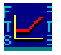Restart to return homeOPTION CALCULATOR:  ANALYTICS

Analytics provides a powerful Value-at-Risk analysis for both the option contract you are currently examining and the underlying asset price.   Thus, its objective is to let you answer the following type of statistical question:

What is the amount such that, with 95% (or 90%, 85% etc.) probability, I will not lose more than that amount over a specified time period?

The specified time period can be 1 day, 1 week, 1 month, ... , maturity.

Answers are provided in both numerical and plotted distributional forms.

To do this, you must enter your estimates for the expected return from the underlying asset and the volatility of the underlying.  For volatility, you can enter the computed implied volatility number from the calculator or your own modification to this number.

For example, suppose the current market price of an IBM (near-the-money) option, with approximately 3 months until maturity, is \$10.375.  Suppose you assess from the current implied volatility that IBM's volatility is 0.65 and suppose, further, that you estimate the expected return from IBM to be 11%.

Analytics will now provide an answer to the question of how much your option premium can decline with 95% likelihood over the next 1 week.

Similarly, you can ask questions of the underlying asset price distribution as well as plotting what the answers to these statistical questions look like when presented as a distribution.

OS Financial Trading System, PO Box 11356, Pittsburgh, PA 15238 USA, Phone 1-800-967-9897, Fax 1-412-967-5958, Email fts@ftsweb.com,